Building graphs with Japanese vocabulary and kanji

This post is a quick and dirty exploration of an aspect of my Japanese learning. The gist behind it is the following: I use a flashcard software called Anki to guide my learning. I use two decks for learning Japanese: one contains my vocabulary and is recognition only, while the other contains my kanji cards and forces me to write the Japanese characters and remember their structure.

In this post, I use the data from both decks combined to create a graph of how the information for a given learning day is connected.

Simple text exploration of the data¶

In :
# necessary imports
from pylab import *
%matplotlib inline
In :
from IPython.html.widgets import interact

Firts, let's read the raw text files containing the data from my decks. jp-kanji contains the kanji I have reviewed today, while jp-vocab contains all the words that I have in my Japanese vocabulary deck.

In :
kanji = genfromtxt('files/kanji.csv', dtype=np.str, usecols=0, delimiter=',')
In :
myvocab = genfromtxt('files/jp_vocab.csv', dtype=np.str, usecols=0, delimiter=',')

The characters I have read so far are encoded using UTF-8. To work with them, I'm converting them to Python unicode.

In :
from codecs import decode
kanji = [decode(k, 'utf-8') for k in kanji]
myvocab = [decode(w, 'utf-8') for w in myvocab]
In :
for k in kanji:
print k,

Let's now write a function that filters my vocabulary list according to whether a given kanji is present in a word or not.

In :
def get_associated_vocab(kanji):
return filter(lambda s: kanji in s, myvocab)
In :
words = get_associated_vocab(kanji)
for w in words:
print w,

We can explore this interactively using the IPython notebook tool interact:

In :
def print_my_vocab(kanji_char):
words = get_associated_vocab(kanji_char)
print "You selected character " + kanji_char
if len(words) != 0:
print "Matches in your vocabulary found!"
for w in words:
print w
else:
print "No match in your vocabulary"
In :
interact(lambda ind: print_my_vocab(kanji[ind]),
ind=(0, len(kanji) - 1))
You selected character 料
Matches in your vocabulary found!

Out:
>

Graphical exploration using NetworkX¶

NetworkX is a toolkit for the study of networks, written in Python. Inspired by this example from the gallery, we can leverage its graphical tools to visualize the data I have analyzed so far.

My aim here is to make a plot with all the kanji reviewed today and link them to their associated vocabulary.

While writing this post, I encountered some problems with the display of Japanese characters. It turns out that this was due to my matplotlib installation and its fonts, that didn't support Japanese characters. I have solved this problem by copying one of my Japanese system fonts within the matplotlib fonts directory located, in my case, at C:\Python27\Lib\site-packages\matplotlib\mpl-data\fonts. A helpful link in this process was http://stackoverflow.com/questions/23197124/display-non-ascii-japanese-characters-in-pandas-plot-legend.

In :
import networkx as nx

Below is a simple test graph that shows how to display a node labelled with a Japanese character.

In :
G = nx.Graph()
nx.draw_networkx(G, font_family='Aozora Mincho')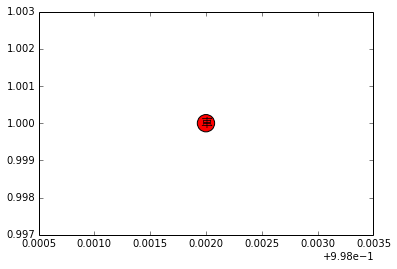This being done, we can move on to more serious things. We create our graph with the following code.

In :
import datetime
In :
figure(figsize=(10, 10))
G = nx.Graph()
today = datetime.date.today()
for k in kanji:
for expr in get_associated_vocab(k):
nx.draw_networkx(G, font_family='Aozora Mincho', font_size=15, node_size=1000)

axis('off');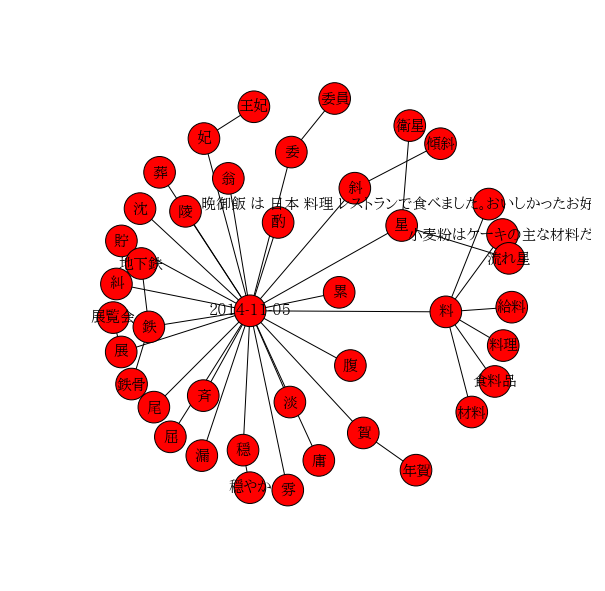This is quite nice, but we can improve it a little bit by using some coloring, inspired by this example.

In :
# creating the graph
G = nx.Graph()
today = datetime.date.today()
for k in kanji:
for expr in get_associated_vocab(k):

# creating the node colors
node_color = []
for node in G:
if node == today:
node_color.append('w')
elif node in kanji:
node_color.append('r')
else:
node_color.append('y')

# plotting
figure(figsize=(10, 10))
pos = nx.spring_layout(G)
nx.draw(G, pos=pos,
with_labels=True,
node_color=node_color,
font_family='Aozora Mincho', font_size=15,
node_size=1000)

axis('off');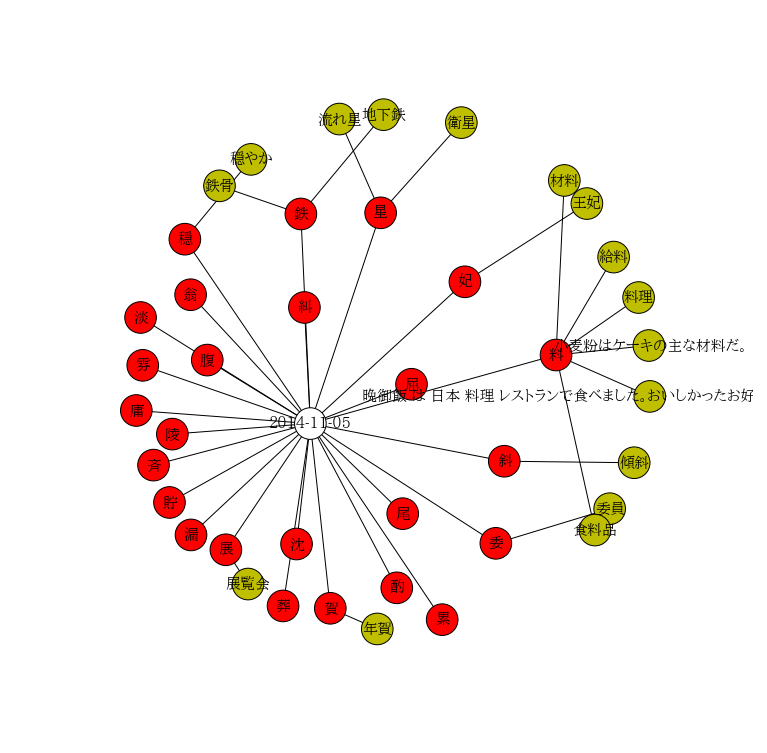While using visualizations like the one above during the last few days, I have noticed that when there are a lot of words and characters to display, the graph gets messy. Therefore, I'll try to produce a more structured version of the previous graph in the next cells.

The graphical output that we have done above consists of two separate steps. The first step is to compute the node positions in the final graph. The second one is drawing using the previous positions.

In the previous cell, we computed the positions of our nodes using nx.spring_layout(G). The positions computed by the spring algorithm are stored in a dictionary:

In :
pos
Out:
{u'\u5983': array([ 0.63895377,  0.67500245]),
u'\u6589': array([ 0.03343059,  0.3185518 ]),
u'\u6599\u7406': array([ 0.97669519,  0.64441187]),
u'\u6c88': array([ 0.31158778,  0.16515432]),
u'\u6de1': array([ 0.0082795 ,  0.60559574]),
u'\u6f0f': array([ 0.10637716,  0.18282049]),
datetime.date(2014, 11, 5): array([ 0.33843229,  0.39933709]),
u'\u8caf': array([ 0.06449885,  0.24593801]),
u'\u6599': array([ 0.81613867,  0.5326309 ]),
u'\u659c': array([ 0.71547702,  0.32610618]),
u'\u661f': array([ 0.47509264,  0.80911579]),
u'\u59d4\u54e1': array([ 0.92043342,  0.23380841]),
u'\u50be\u659c': array([ 0.96810298,  0.32317835]),
u'\u6d41\u308c\u661f': array([ 0.39479764,  0.9914399 ]),
u'\u7d66\u6599': array([ 0.92811008,  0.72305464]),
u'\u7d2f': array([ 0.59474906,  0.01805415]),
u'\u5e74\u8cc0': array([ 0.46854098,  0.        ]),
u'\u5eb8': array([ 0.        ,  0.42477971]),
u'\u5c3e': array([ 0.51802025,  0.22415128]),
u'\u7fc1': array([ 0.10560111,  0.64988196]),
u'\u8cc0': array([ 0.37718882,  0.04015944]),
u'\u9244': array([ 0.32000005,  0.80690719]),
u'\u5c48': array([ 0.53514343,  0.47579271]),
u'\u885b\u661f': array([ 0.63198603,  0.98454265]),
u'\u914c': array([ 0.5064797 ,  0.07996336]),
u'\u7a4f': array([ 0.09442873,  0.75785548]),
u'\u5c55\u89a7\u4f1a': array([ 0.21722116,  0.08730433]),
u'\u5c55': array([ 0.17416823,  0.15397226]),
u'\u98df\u6599\u54c1': array([ 0.89176716,  0.19228279]),
u'\u5730\u4e0b\u9244': array([ 0.48069608,  1.        ]),
u'\u738b\u5983': array([ 0.8761446 ,  0.82716321]),
u'\u9244\u9aa8': array([ 0.16174776,  0.86162271]),
u'\u5c0f\u9ea6\u7c89\u306f\u30b1\u30fc\u30ad\u306e\u4e3b\u306a\u6750\u6599\u3060\u3002': array([ 0.99668281,  0.55111055]),
u'\u6750\u6599': array([ 0.83223969,  0.87208056]),
u'\u6669\u5fa1\u98ef \u306f \u65e5\u672c \u6599\u7406 \u30ec\u30b9\u30c8\u30e9\u30f3\u3067\u98df\u3079\u307e\u3057\u305f\u3002\u304a\u3044\u3057\u304b\u3063\u305f\u304a\u597d\u307f\u713c\u304d\u98df\u3079\u307e\u3057\u305f\u3002

': array([ 0.99848304, 0.45215401]), u'\u846c': array([ 0.28533294, 0.0447483 ]), u'\u96f0': array([ 0.01251561, 0.51255702]), u'\u7a4f\u3084\u304b': array([ 0.22307882, 0.91305033]), u'\u9675': array([ 0.07011004, 0.37885549]), u'\u8179': array([ 0.13798064, 0.52279467]), u'\u7cfe': array([ 0.3268805 , 0.62509542]), u'\u59d4': array([ 0.69909341, 0.16646906])}

My plan in the next cells is to compute the positions by myself instead of using the built-in algorithm.

In :
# creating the graph
G = nx.Graph()
today = datetime.date.today()

for k in kanji:
for expr in get_associated_vocab(k):

# creating the node colors
node_color = []
for node in G:
if node in kanji:
node_color.append('r')
else:
node_color.append('y')

# computing positions
pos = {}
r1 = 0.3 * len(kanji) / 100.
r2 = r1 / 4.
theta = linspace(0, 2 * pi, len(kanji), endpoint=False)
for ind, k in enumerate(kanji):
pos[k] = r1 * array([cos(theta[ind]), sin(theta[ind])])
words = get_associated_vocab(k)
alpha = linspace(0, 2 * pi, len(words), endpoint=False) + theta[ind] + pi / 4 + (pi / 4) * (ind % 2)
for ind2, w in enumerate(words):
if not w in pos:
pos[w] = r1 * array([cos(theta[ind]), sin(theta[ind])]) + r2 * array([cos(alpha[ind2]), sin(alpha[ind2])])

# plotting
figure(figsize=(10, 10))
nx.draw(G, pos=pos,
with_labels=True,
node_color=node_color,
font_family='Aozora Mincho', font_size=15,
node_size=1000)

axis('off');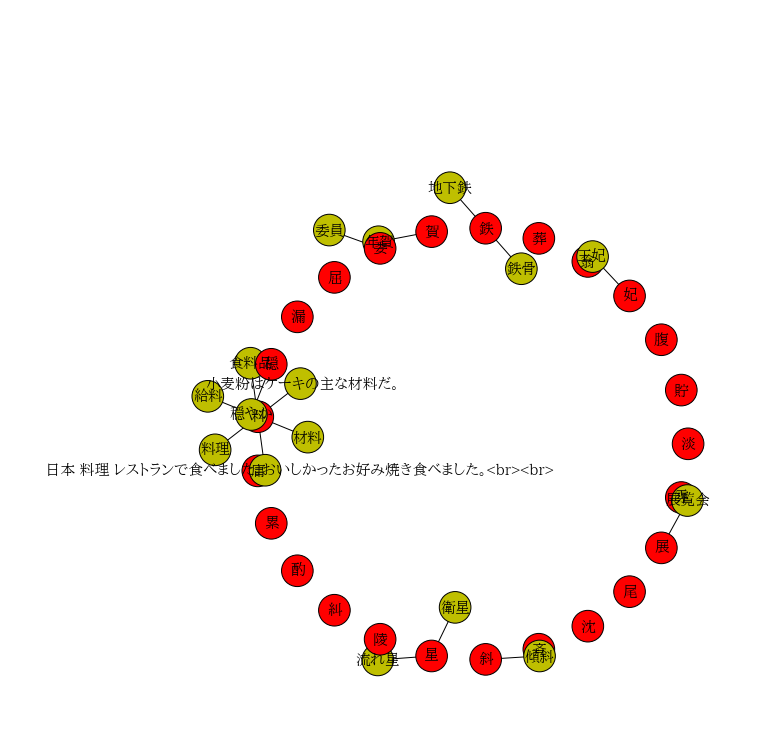This is still not satisfying. So based on the above layout, we will build a small particle simulation. First, we define a particle class that will be able to model repulsive forces, based on its mass, inertia, and spring-like behaviour with a set of "neighbours" to which the particle is linked.

In :
class particle(object):
def __init__(self, name, pos, fixed=False, mass=1., neighbours=[], stiffness=0.1):
# inits a particle with a given mass and a given stiffness linkind it to its neighbours
self.pos = pos
self.mass = mass
self.fixed = fixed
self.neighbours = neighbours
self.speed = zeros((2))
self.stiffness = stiffness
self.name = name
self.external_force = zeros((2))

def move(self, dt):
# moves the particle for a timestep dt
self.speed += dt/self.mass * self.external_force
self.pos += self.speed * dt

def compute_spring_force(self):
# computes the force exerted upon the node by its neighbours
force_vector = zeros((2))
for neighbour in self.neighbours:
force_vector += self.stiffness * (particles[neighbour].pos - self.pos)
return force_vector

Next, we can code the simulation. First, we build the list of nodes we want to work with.

In :
pos = nx.spring_layout(G)
particles = {}
for k in kanji:
fixed = True
neighbours = get_associated_vocab(k)
particles[k] = particle(k, pos[k], fixed=True, mass=len(neighbours) + 1, neighbours=neighbours)
for neigh in neighbours:
particles[neigh] = particle(neigh, pos[neigh], fixed=False, neighbours=[k])
In :
def get_pos_dict():
pos = dict(zip(particles.keys(), [particles[k].pos for k in particles]))
pos[datetime.date(2014, 11, 5)] = [0., 0.]
return pos
In :
def plot_current_particles():
figure(figsize=(10, 10))
nx.draw(G, pos=get_pos_dict(),
with_labels=True,
node_color=node_color,
font_family='Aozora Mincho', font_size=15,
node_size=1000)

Next, we build the iteration steps:

In :
def iterate(dt):
for current_p in particles:
external_force = zeros((2))
for other_p in particles:
if current_p != other_p:
# sum repulsive force
external_force -= particles[other_p].mass / (particles[current_p].pos - particles[other_p].pos) ** 2 * (particles[current_p].pos - particles[other_p].pos)
external_force += particles[current_p].compute_spring_force()
particles[current_p].external_force = external_force
for current_p in particles:
particles[current_p].move(dt)

Let's move this graph.

In :
iterate(10)
plot_current_particles()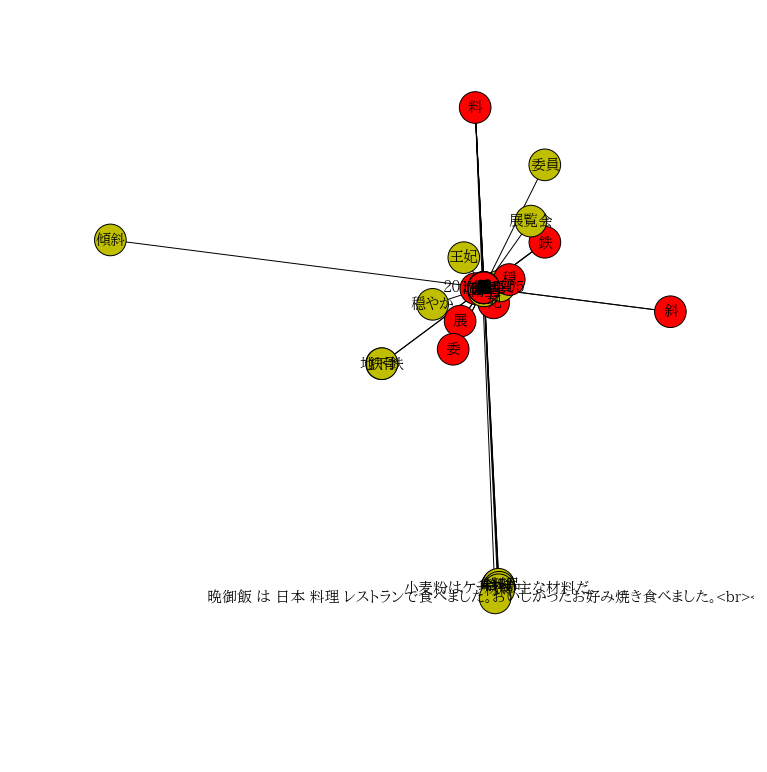In :
def iterate_and_plot(dt):
iterate(dt)
figure(figsize=(10, 10))
nx.draw(G, pos=get_pos_dict(),
with_labels=True,
node_color=node_color,
font_family='Aozora Mincho', font_size=15,
node_size=1000)
axis('off');
In :
from IPython.html.widgets import ButtonWidget
from IPython.display import display, clear_output
In :
b = ButtonWidget(description="iteration")
display(b)
b.on_click(lambda b: iterate_and_plot(0.1))
In :
iterate_and_plot(0.02)
---------------------------------------------------------------------------
NetworkXError                             Traceback (most recent call last)
in ()
----> 1 iterate_and_plot(0.02)

in iterate_and_plot(dt)
7         node_color=node_color,
8         font_family='Aozora Mincho', font_size=15,
----> 9         node_size=1000)
10     axis('off');

C:\Python27\lib\site-packages\networkx\drawing\nx_pylab.pyc in draw(G, pos, ax, hold, **kwds)
129         plt.hold(h)
130     try:
--> 131         draw_networkx(G, pos=pos, ax=ax, **kwds)
132         ax.set_axis_off()
133         plt.draw_if_interactive()

C:\Python27\lib\site-packages\networkx\drawing\nx_pylab.pyc in draw_networkx(G, pos, with_labels, **kwds)
262         pos = nx.drawing.spring_layout(G)  # default to spring layout
263
--> 264     node_collection = draw_networkx_nodes(G, pos, **kwds)
265     edge_collection = draw_networkx_edges(G, pos, **kwds)
266     if with_labels:

C:\Python27\lib\site-packages\networkx\drawing\nx_pylab.pyc in draw_networkx_nodes(G, pos, nodelist, node_size, node_color, node_shape, alpha, cmap, vmin, vmax, ax, linewidths, label, **kwds)
373         xy = numpy.asarray([pos[v] for v in nodelist])
374     except KeyError as e:
--> 375         raise nx.NetworkXError('Node %s has no position.'%e)
376     except ValueError:
377         raise nx.NetworkXError('Bad value in node positions.')

NetworkXError: Node datetime.date(2014, 11, 5) has no position.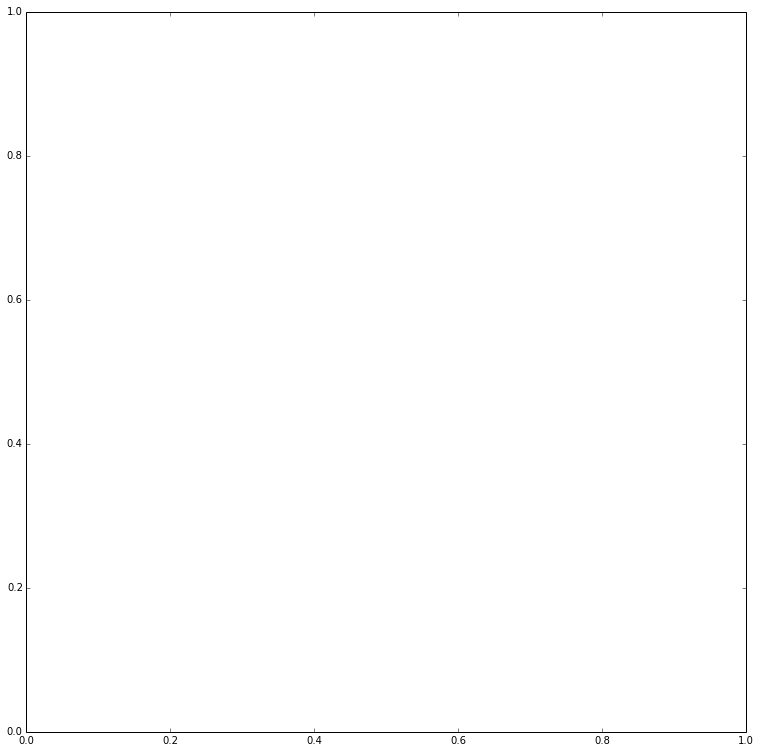In :
particles[u"星"].move(1)
particles[u"星"].pos
Out:
array([             nan,  -1.10393199e+11])
In [ ]:
pos
In [ ]:

That's it! A brand new tool for visualizing the connections between your kanji deck and your vocabulary!